Courses
Courses for Kids
Free study material
Free LIVE classes
More

# Subtraction of NumbersLIVE
Join Vedantu’s FREE Mastercalss

## Introduction to Subtraction of Large Numbers

Subtraction is an operation in which we take something out of a collection of objects. For example, taking out 4 mangoes from 7 mangoes. It is an arithmetic operation between two numbers. It has great significance in mathematics since it forms the base for our mathematical understanding. Let us learn how to subtract the numbers.

### What is Subtraction?

It is an arithmetic operation that signifies the removal of several objects from a collection. In other words, suppose you have 5 Apples, and you ate 3 of them, then you are left with only 2 Apples, this course of an arithmetic operation is called subtraction. This is the most basic form of math we use daily. From shopping to handling a business.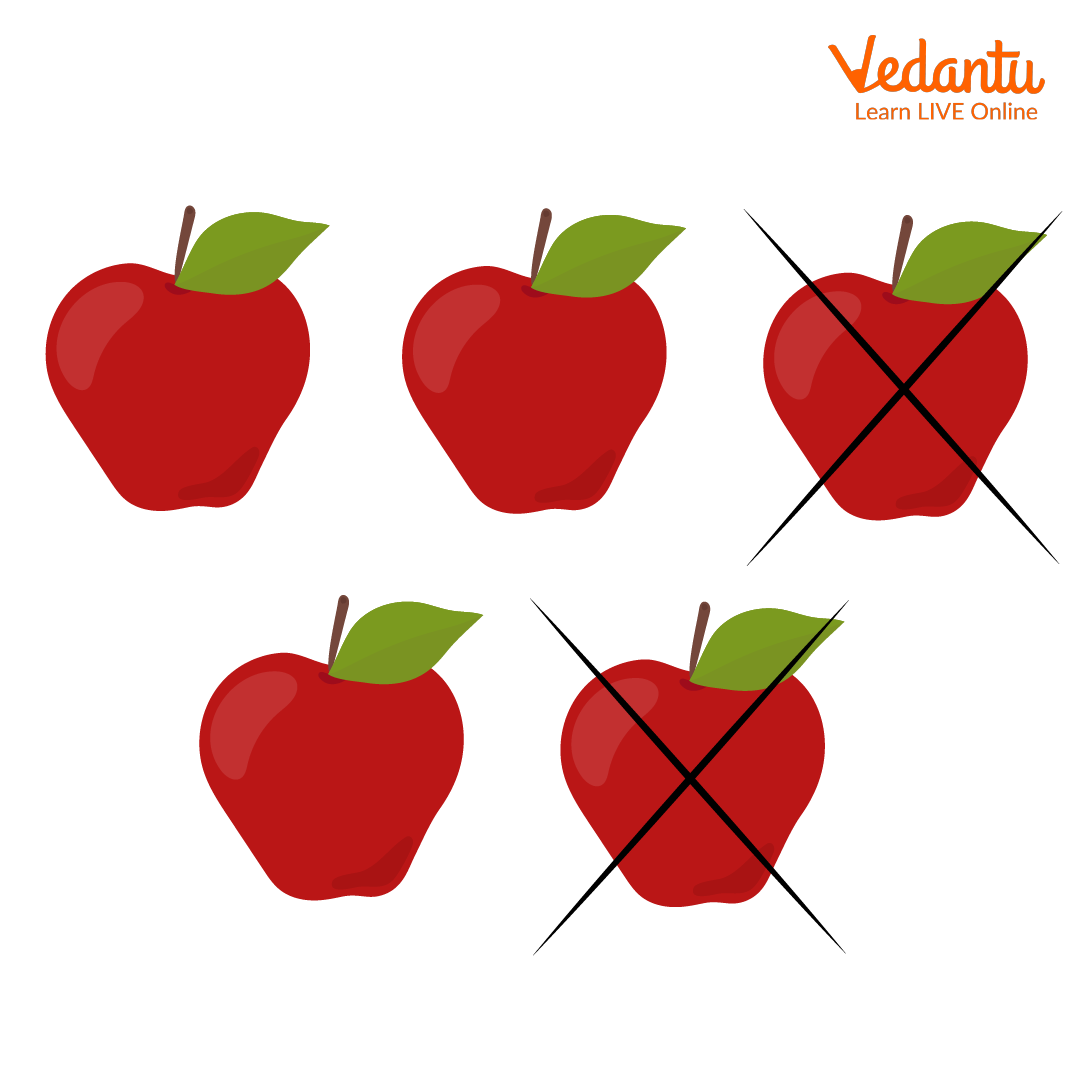Subtraction

### Why is Subtraction Important?

Subtraction is the backbone of the number system. We use it on an everyday basis. Suppose you go to the supermarket to buy vegetables, and the potatoes cost 20 rupees per kilogram, you buy a total of 4 kilograms of potatoes, and you have a total of 110 rupees, then how much are you left with? The answer is simple, you have to pay $20 \times 4 = 80$ rupees, and you have 110 rupees. After paying 80 rupees to the shopkeeper your balance amount is $110 - 80 = 30$ rupees. Don’t fret. We will learn how to subtract two numbers in just a moment.

### How to Subtract Two Numbers?

We will learn how to subtract two numbers. These are the steps to subtracting two numbers.

1. Write down the larger the number. Let's take an example where we want to subtract 17 from 32.

2. Write the smaller number right below, the larger number. Do not forget to line up the ones, tens, and hundreds,... Places. In our case 7 is directly below 2 and 1 is directly below 3.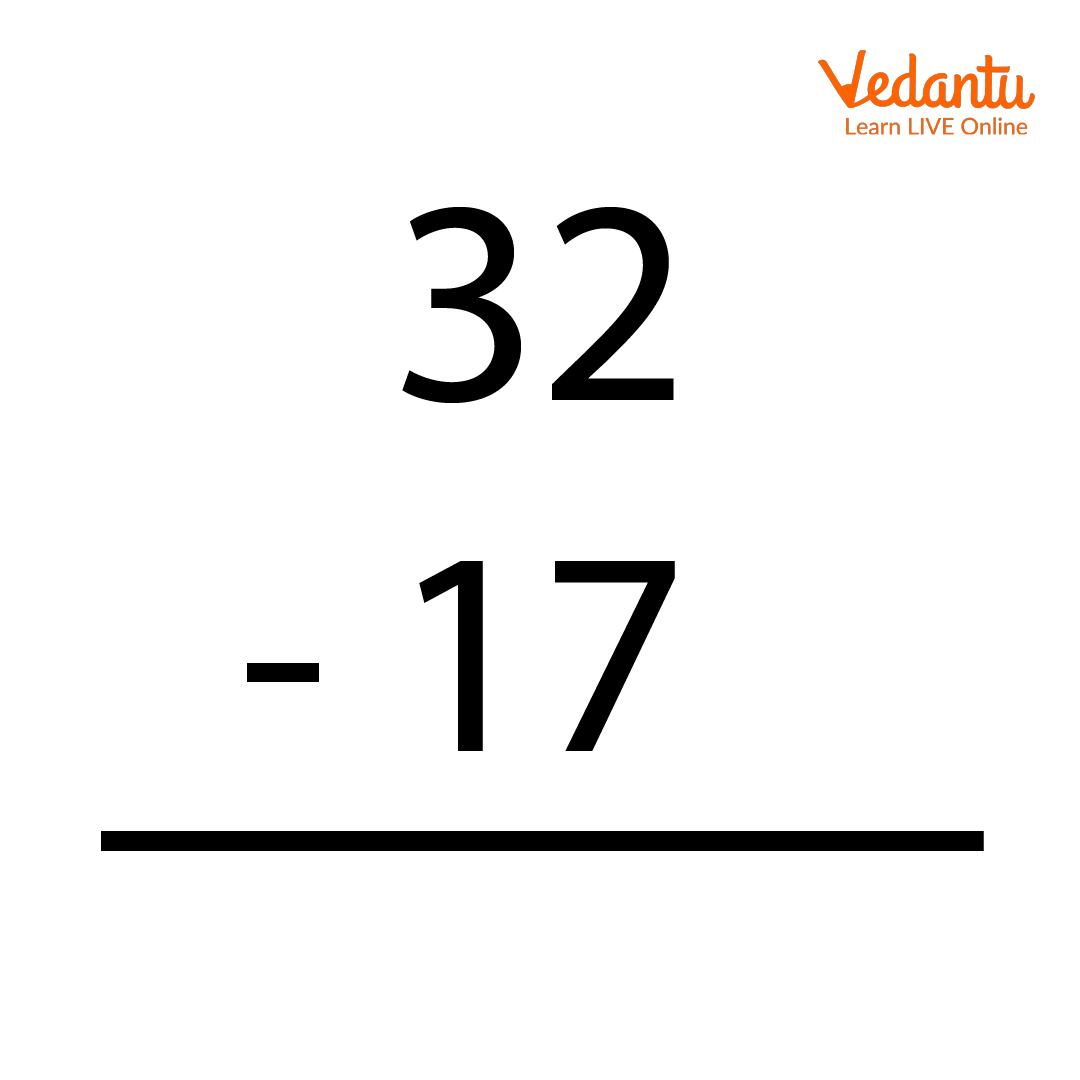Subtraction

1. Start subtracting the numbers from right to left. If the digit above is greater than the lower one, we do simple subtraction, and if the number above is lesser than the lower one, we borrow 1 from the digit next to the upper one on the left, cross off the digit and make it 1 less than it was. For example, in our case, we have to subtract 7 from 2, so we borrow 1 from 3, and it makes 12, so instead of subtracting 7 from 2, now we have to subtract 7 from 12, and we also cross off 3 and write 2 in place of it. So $12 - 7 = 5$.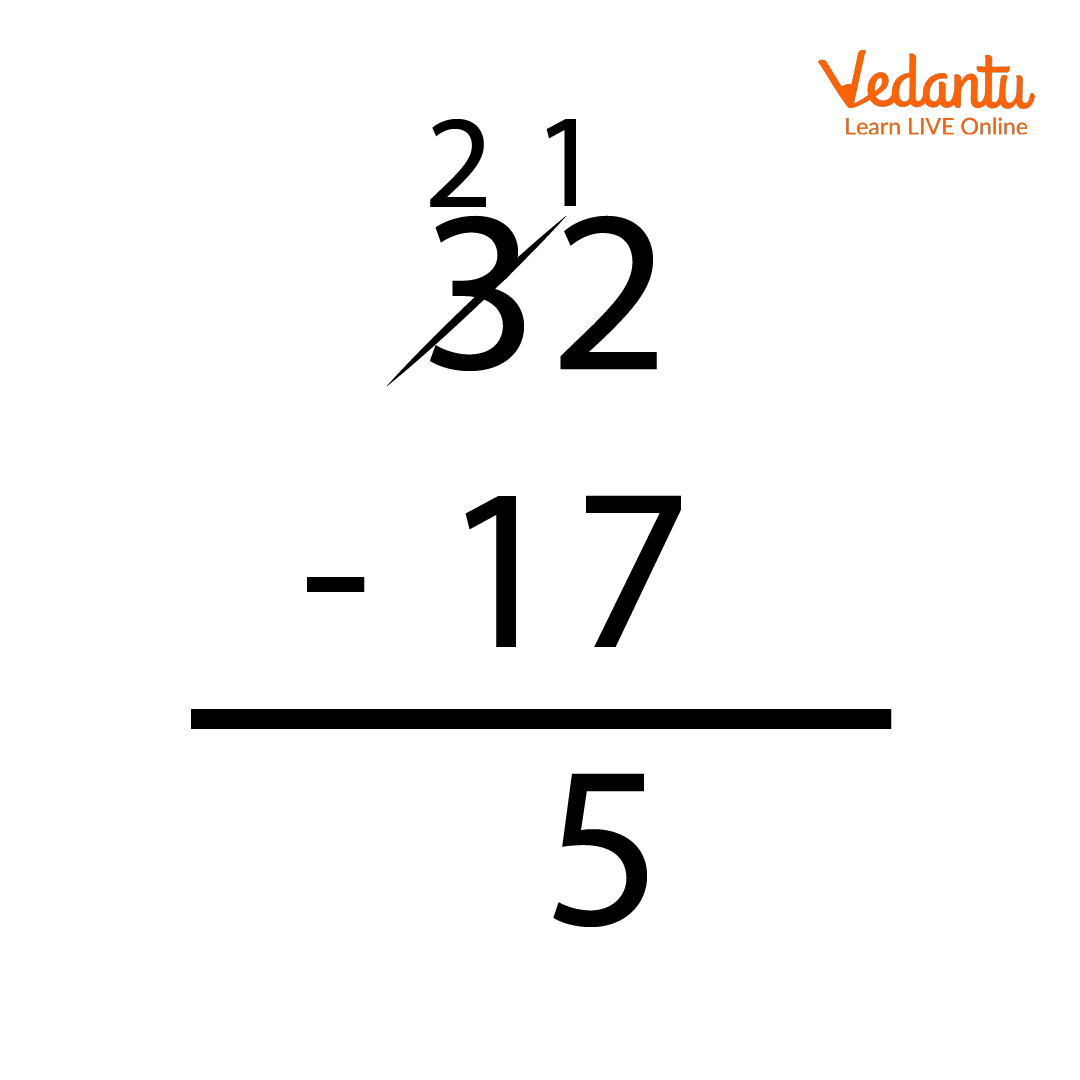Subtraction

1. Now we subtract the tens place digit, which is $2 - 1 = 1$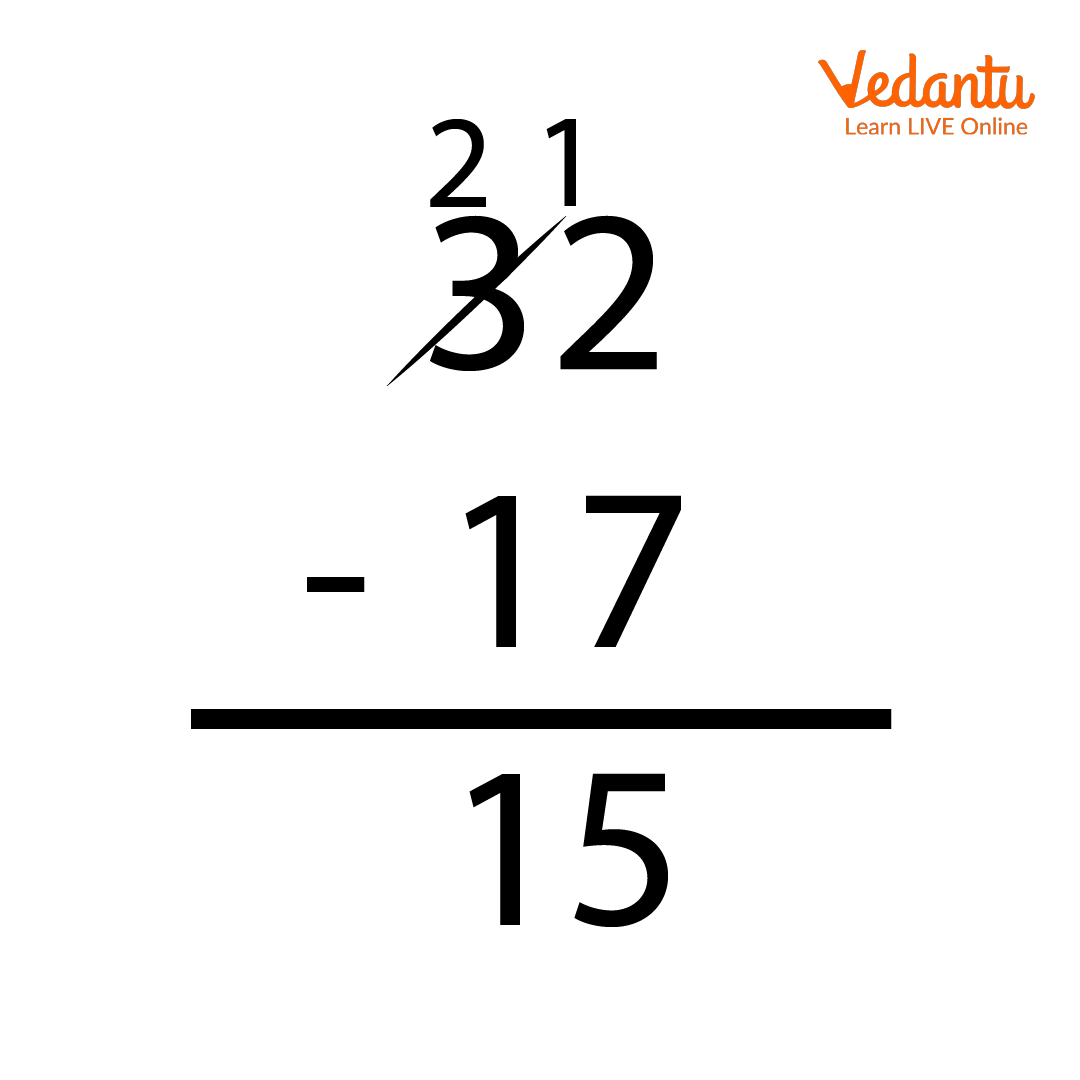Subtraction

1. Therefore our final answer is 15. Check the answer, $17 + 15 = 32$.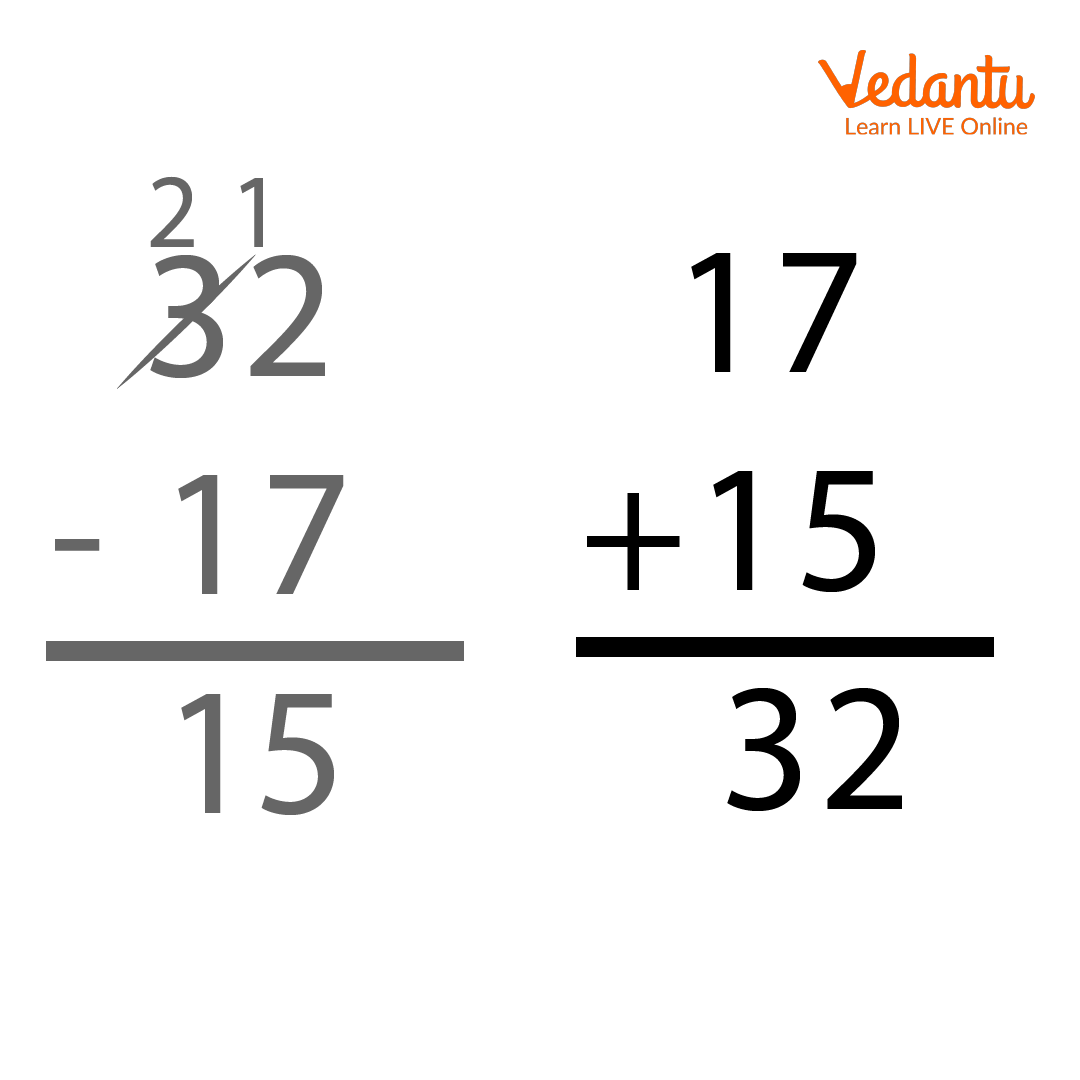Subtraction

### Important Notes

Here are some of the important notes.

• If a smaller number is subtracted from a big number, then the answer is always a positive number.

• If a bigger number is subtracted from a small number, then the answer is always a negative number.

• If a number is subtracted from itself, we get 0 as the answer. For example, 7 subtracted from 7 is 0, and 863 subtracted from 863 is 0.

Great! You now understand what subtraction of numbers is. You have learned how to subtract two numbers and how to do it step by step. Apart from this, you have also learned some important facts about subtraction. Now subtract the following numbers given in the worksheet below:

### Solved Examples

1. Sophie has 27 pens, she sold 15 pens out of those 27 pens. How many pens are left at Sophie?

Ans: Sophia has 27 pens, and she sold 15 pens; we can see that the problem asks for the subtraction of two numbers. Here we have to subtract 15 out of 27 to find the number of chocolates left to Sophia. So we have $27 - 15$. $7 - 5$ is 2 and $2 - 1$ is 1. Hence $27 - 15$ is 12.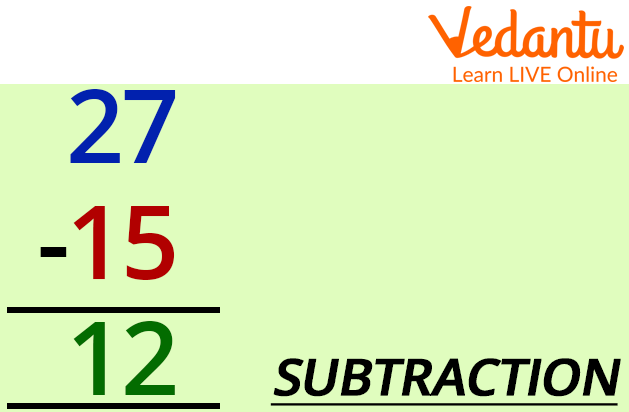Subtraction

2. It's your birthday, and you have brought 100 chocolates. You have 5 friends; you decided to give them 10, 10,10,20, and 20 chocolates. How many chocolates are you left with?

Ans: This problem asks for two things, first, we have to calculate how many total chocolates has he given to his friends then we will have to find the number of chocolates left by him. So the total number of chocolates he has given is$10 + 10 + 10 + 20 + 20 = 70$. Now we have to subtract this number from the total number of chocolates to see how many chocolates are still left. So we have to subtract 70 from 100. 0 - 0 is 0 and then we have $0 - 7$ in the tens place. 0 is less than 7, so we borrow 1 from 1 and then subtract 7. So $10 - 7 = 3$ hence $100 - 70 = 30$. Thus, only 30 chocolates are left for you.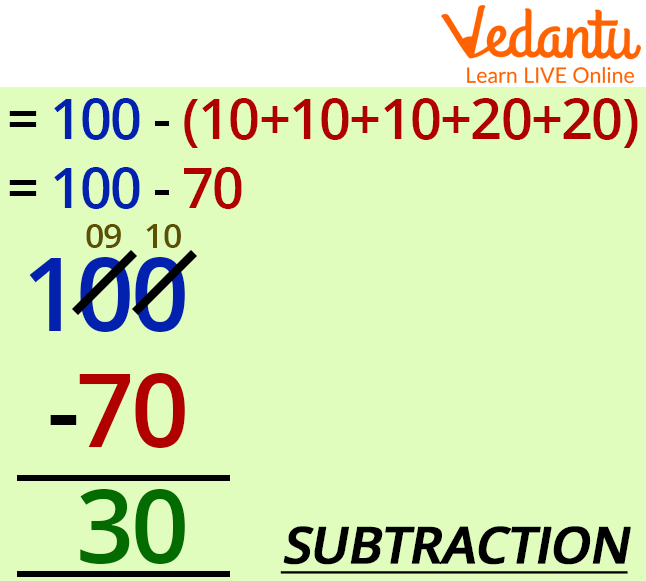Subtraction

### Conclusion

Subtraction is very vital in our daily life. And it is the very base of mathematical reasoning. We use it in many fields of mathematics, as well as in our daily lives. It is a fundamental arithmetic operation, yet the most important is just like addition, multiplication, and division.

Last updated date: 29th Sep 2023
Total views: 70.2k
Views today: 2.70k

## FAQs on Subtraction of Numbers

1. What do we get when we subtract any number from itself?

When subtracting any number from itself, we get a 0. For example, let's say you have 5 candies, and you ate all of them; what are you left with? Yes, 0 candies.

2. What is the result when we subtract 40 out of -50?

When we subtract 40 from $- 50$, we get $- 90$. [Why?] Suppose you borrowed 50 rupees from your sister and you borrowed 40 more rupees. Then how much money is borrowed in total? Yes, 90 rupees were borrowed. Hence it is -90.

3. Can we subtract two negative numbers?

Yes, we can subtract two negative numbers. When we subtract a negative number we make it positive. For example, $[ - 40] - [ - 30] = [ - 40] + 30 = - 10$.

4. What are the terms of subtraction called?

The terms of the subtraction are called minuend and subtrahend. And the result is called the difference. The number from which we subtract is called minuend, and the number which is subtracted is called subtrahend.

5. What are the three common properties of subtraction?

The three common properties of subtraction are Associative property, Distributive property, and Commutative property.